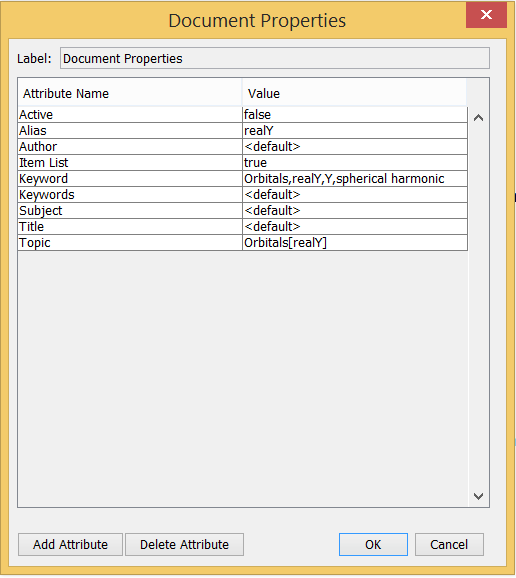## dharr

Dr. David Harrington

## 3020 Reputation

17 years, 303 days
University of Victoria
Professor or university staff

## Social Networks and Content at Maplesoft.comI am a professor of chemistry at the University of Victoria, BC, Canada, where my research areas are electrochemistry and surface science. I have been a user of Maple since about 1990.

## Showing quadratic forms are nonnegative...

Maple
 > restart;
 > with(LinearAlgebra):

Recently @Nour asked (in part) if Maple can simplify 2*(x^2 - x*y - x*z + y^2) to (x - y)^2 + (y - z)^2 + (z - x)^2. User @vv pointed out that a sum of squares form is not unique. I'm not sure if @Nour's intent was to show that this was always non-negative, but even if the sum of squares form is not unique, it does help to demonstrate that the expression will be nonnegative for all real x,y,z. This reminded me that I have over the years had cases where I wanted to know if a quadratic form (multivariate polynomial with all terms of degree 2) would always be non-negative (a quadratic form will be zero if all variables are zero, so we know it won't always be positive). I have typically done this by hand in the following way, which I demonstrate for the simpler case

 > p1:=2*x^2-3*x*y+4*y^2;We make a (column) vector of the variables, v = [x,y] and then construct the symmetric Matrix A for which Transpose(v).A.v is the required expression. For the squared term 2*x^2, the coefficient 2 is put in the diagonal entry A[1,1] and likewise 4*y^2 leads to A[2,2] = 4. The term -3*x*y comes from the sum A[1,2]+A[2,1] and so half of the coefficient -3 goes to each of A[2,1] and A[1,2]. The Matrix is therefore

 > A:=Matrix(2,2,[2,-3/2,-3/2,4]);v:=Vector([x,y]):simplify(v^+.A.v);The Matrix is found to be positive definite (positive eigenvalues), which means that the quadratic form is positive for all non-zero real vectors v.

 > IsDefinite(A,query='positive_definite');evalf(Eigenvalues(A));One way to get the sum of squares form is to use the Cholesky decomposition (for positive definite or semidefinite matrices), which yields A = L.Transpose(L) = L.I.Transpose(L), and then note that Transpose(L).v is a change of variables to a diagonal quadratic form

 > L:=LUDecomposition(A,method='Cholesky'); L.L^+;so we have

 > v2:=L^+.v; v2^+.v2; map(simplify,%);and we have rewritten the quadratic form in an explicitly nonnegative form.

Another way to the sum of squares would be via the othogonal similarity A = U.Lambda.Transpose(U), where U is an orthogonal matrix of eigenvectors and Lambda is diagonal with eigenvalues on the diagonal. This form works even if the matrix is not positive (semi)definite.

In this simple case, Maple has some other ways of showing that the quadratic form is non-negative for real x,y

 > Student[Precalculus]:-CompleteSquare(p1,x);Without assumptions, is correctly decides p1 is not always nonnegative, and an example of this for complex x and y is easily found. With assumptions, is fails.

 > is(p1,nonnegative); eval(p1,{x=I,y=I}); is(p1,nonnegative) assuming real;At least in Maple 2017, solve suggests any x and y will do, which is not quite right

 > solve(p1>=0);In the next worksheet, I implement this method in a general procedure IsQformNonNeg, which works as well as IsDefinite does. For most cases of interest (integer or rational coefficients) there are no problems. For floating point coefficients, as always, numerical roundoff issues arise, and I dealt with this by giving warnings. (Rank is more robust in these cases than IsDefinite, but since this case can be better dealt with by converting floats to rationals, I haven't worked too hard on this - perhaps IsDefinite will be improved.)

The original example 2*(x^2 - x*y - x*z + y^2)  is dealt with as an example in the next worksheet

 >

## Creating Help - using HelpTools...

Maple

@ianmccr posted here about making help for a package using makehelp. Here I show how to do this with the HelpTools package.

The attached worksheet shows how to create the help database for the Orbitals package available at the Applications Center or in the Maple Cloud. The help pages were created as worksheets - start using an existing help page as a model - use View/Open Page As Worksheet and then save from there. The topics and other information are entered by adding Attributes under File/Document Properties - for example for the realY help page these are:Active=false means a regular help page; Active=true means an example worksheet.

There may be several aliases, for example the cartesion help page also describes the fullcartesian command and so Alias is: Orbitals[fullcartesian],cartesian,fullcartesian and Keyword is: Orbitals,cartesian,fullcartesian

Once a worksheet is created for each help page they are assembled into the help database with the attached file. More information is in the attached file

Orbitals_Make_help_database.mw

 Page 1 of 1
﻿